# CD Get Sampling Time from Model VI

LabVIEW 2018 Control Design and Simulation Module Help

Edition Date: March 2018

Part Number: 371894J-01

»View Product InfoDownload Help (Windows Only)

Owning Palette: Model Information VIs

Requires: Control Design and Simulation Module

Obtains the sampling time of the system. The sampling time is zero for continuous systems and greater than zero for discrete systems. Wire data to the State-Space Model input to determine the polymorphic instance to use or manually select the instance.

Use the pull-down menu to select an instance of this VI.

 Select an instance CD Get Sampling Time from Model (State-Space Model)CD Get Sampling Time from Model (Transfer Function)CD Get Sampling Time from Model (Zero-Pole-Gain)

## CD Get Sampling Time from Model (State-Space Model)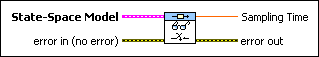State-Space Model contains a mathematical representation of and information about the system for which this VI obtains sampling time.error in describes error conditions that occur before this node runs. This input provides standard error in functionality.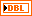Sampling Time is the sampling time this VI associates with the system model. Sampling Time defines whether the model represents a continuous-time system or a discrete-time system. If the model represents a continuous-time system, Sampling Time equals 0. If the model represents a discrete-time system, Sampling Time is greater than 0 and equal to the sampling rate, in seconds, of the discrete system. A value of -1 indicates that the sampling time is irrelevant.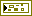error out contains error information. This output provides standard error out functionality.

## CD Get Sampling Time from Model (Transfer Function)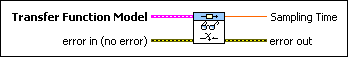Transfer Function Model contains a mathematical representation of and information about the system for which this VI obtains sampling time.error in describes error conditions that occur before this node runs. This input provides standard error in functionality.Sampling Time is the sampling time this VI associates with the system model. Sampling Time defines whether the model represents a continuous-time system or a discrete-time system. If the model represents a continuous-time system, Sampling Time equals 0. If the model represents a discrete-time system, Sampling Time is greater than 0 and equal to the sampling rate, in seconds, of the discrete system. A value of -1 indicates that the sampling time is irrelevant.error out contains error information. This output provides standard error out functionality.

## CD Get Sampling Time from Model (Zero-Pole-Gain)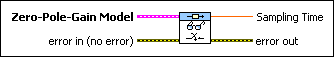Zero-Pole-Gain Model contains a mathematical representation of and information about the system for which this VI obtains sampling time.error in describes error conditions that occur before this node runs. This input provides standard error in functionality.Sampling Time is the sampling time this VI associates with the system model. Sampling Time defines whether the model represents a continuous-time system or a discrete-time system. If the model represents a continuous-time system, Sampling Time equals 0. If the model represents a discrete-time system, Sampling Time is greater than 0 and equal to the sampling rate, in seconds, of the discrete system. A value of -1 indicates that the sampling time is irrelevant.error out contains error information. This output provides standard error out functionality.